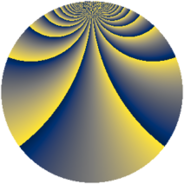# Properties

 Label 1380.2.buLevel $1380$ Weight $2$ Character orbit 1380.bu Rep. character $\chi_{1380}(77,\cdot)$ Character field $\Q(\zeta_{44})$ Dimension $960$ Sturm bound $576$

# Related objects

## Defining parameters

 Level: $$N$$ $$=$$ $$1380 = 2^{2} \cdot 3 \cdot 5 \cdot 23$$ Weight: $$k$$ $$=$$ $$2$$ Character orbit: $$[\chi]$$ $$=$$ 1380.bu (of order $$44$$ and degree $$20$$) Character conductor: $$\operatorname{cond}(\chi)$$ $$=$$ $$345$$ Character field: $$\Q(\zeta_{44})$$ Sturm bound: $$576$$

## Dimensions

The following table gives the dimensions of various subspaces of $$M_{2}(1380, [\chi])$$.

Total New Old
Modular forms 6000 960 5040
Cusp forms 5520 960 4560
Eisenstein series 480 0 480

## Trace form

 $$960q - 4q^{3} + O(q^{10})$$ $$960q - 4q^{3} + 8q^{13} + 8q^{15} - 40q^{25} + 8q^{27} - 16q^{31} - 18q^{33} + 96q^{37} + 24q^{43} + 16q^{51} + 8q^{55} - 16q^{57} + 120q^{61} - 12q^{63} - 8q^{67} + 52q^{75} + 88q^{81} - 8q^{85} - 56q^{87} - 48q^{91} - 8q^{93} + 132q^{97} + O(q^{100})$$

## Decomposition of $$S_{2}^{\mathrm{new}}(1380, [\chi])$$ into newform subspaces

The newforms in this space have not yet been added to the LMFDB.

## Decomposition of $$S_{2}^{\mathrm{old}}(1380, [\chi])$$ into lower level spaces

$$S_{2}^{\mathrm{old}}(1380, [\chi]) \cong$$ $$S_{2}^{\mathrm{new}}(345, [\chi])$$$$^{\oplus 3}$$$$\oplus$$$$S_{2}^{\mathrm{new}}(690, [\chi])$$$$^{\oplus 2}$$